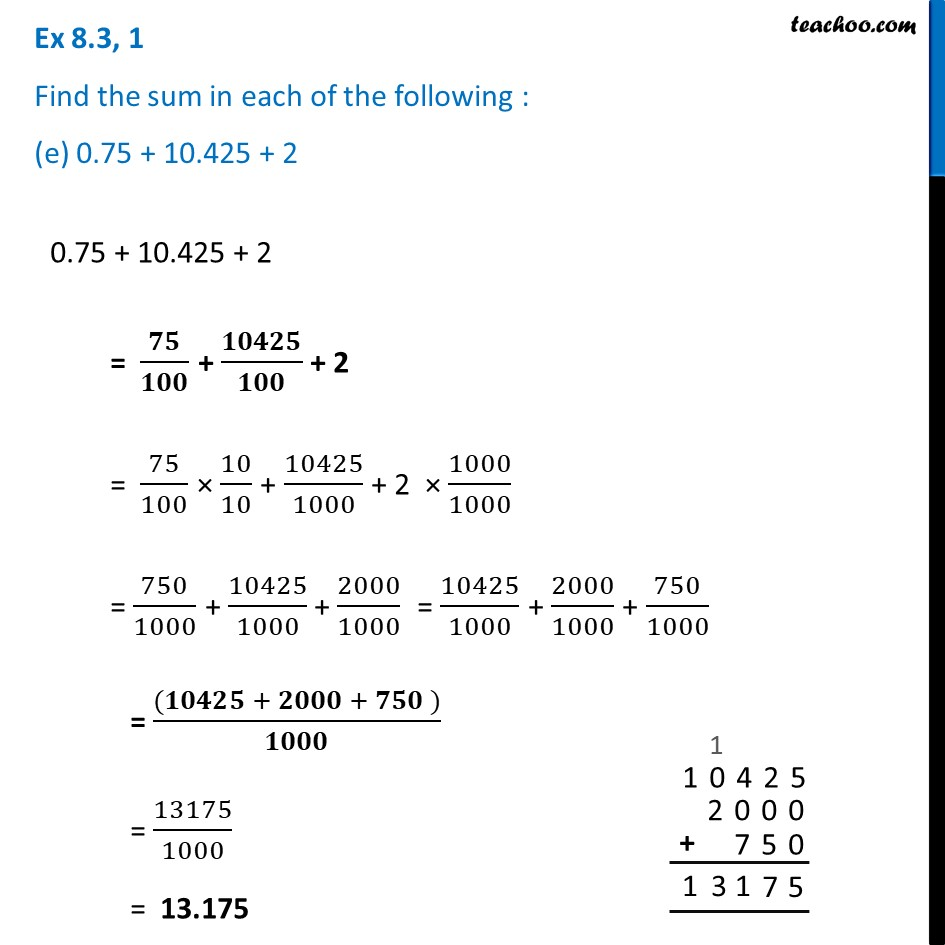Ex 8.3

Chapter 8 Class 6 Decimals
Serial order wiseLearn in your speed, with individual attention - Teachoo Maths 1-on-1 Class

### Transcript

Ex 8.3, 1 Find the sum in each of the following : (e) 0.75 + 10.425 + 20.75 + 10.425 + 2 = 𝟕𝟓/𝟏𝟎𝟎 + 𝟏𝟎𝟒𝟐𝟓/𝟏𝟎𝟎 + 2 = 75/100 × 10/10 + 10425/1000 + 2 × 1000/1000 = 750/1000 + 10425/1000 + 2000/1000 = 10425/1000 + 2000/1000 + 750/1000 = ((𝟏𝟎𝟒𝟐𝟓 + 𝟐𝟎𝟎𝟎 + 𝟕𝟓𝟎 ))/𝟏𝟎𝟎𝟎 = 13175/1000 = 13.175